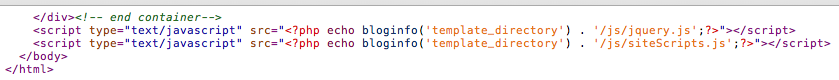##帐号 自动登录 找回密码 密码 注册

# 给JavaScript初学者的24条最佳实践

2013-12-12 13:49| 发布者: 红黑魂| 查看: 2741| 评论: 0|来自: 伯乐在线

## 1.使用 === 代替 ==

JavaScript 使用2种不同的等值运算符：===|!== 和 ==|!=，在比较操作中使用前者是最佳实践。

“如果两边的操作数具有相同的类型和值，===返回true，!==返回false。”——《JavaScript：语言精粹》

## 3.省略未必省事

 12 `if``(someVariableExists)  ``   ``x = ``false`

 123 `if``(someVariableExists)  ``   ``x = ``false` `   ``anotherFunctionCall();`

 1234 `if``(someVariableExists) {  ``   ``x = ``false``;  ``   ``anotherFunctionCall();  ``}`

 1234 `if``(someVariableExists) {  ``   ``x = ``false``;  ``}  ``anotherFunctionCall();`

 1 `if``(2 + 2 === 4) ``return` `'nicely done'``;`

## 4.使用JSLint

JSLint是由大名鼎鼎的道格拉斯（Douglas Crockford）编写的调试器。简单的将你的代码粘贴进JSLint中，它会迅速找出代码中明显的问题和错误。

“JSLint扫面输入的源代码。如果发现一个问题，它返回一条描述问题和一个代码中的所在位置的消息。问题并不一定是语法错误，尽管通常是这样。JSLint还会查看一些编码风格和程序结构问题。这并不能保证你的程序是正确的。它只是提供了另一双帮助发现问题的眼睛。”——JSLing 文档

## 5.将脚本放在页面的底部12345 `

And now you know my favorite kinds of corn. <``/p``>  ``

## 6.避免在For语句内声明变量

 12345 `for``(var i = 0; i < someArray.length; i++) {  ``   ``var container = document.getElementById(``'container'``);  ``   ``container.innerHtml += ``'my number: '` `+ i;  ``   ``console.log(i);  ``}`

 12345 `var container = document.getElementById(``'container'``);  ``for``(var i = 0, len = someArray.length; i < len;  i++) {  ``   ``container.innerHtml += ``'my number: '` `+ i;  ``   ``console.log(i);  ``}`

## 7.构建字符串的最优方法

 12 `var arr = [``'item 1'``, ``'item 2'``, ``'item 3'``, ...];  ``var list = ``'
• '` `+ arr.``join``(``'
• '``) + ``'
'``;`

## 8.减少全局变量

只要把多个全局变量都整理在一个名称空间下，拟将显著降低与其他应用程序、组件或类库之间产生糟糕的相互影响的可能性。——Douglas Crockford

 123456 `var name = ``'Jeffrey'``;  ``var lastName = ``'Way'``;  ` `function` `doSomething() {...}  ` `console.log(name); ``//` `Jeffrey -- 或 window.name`

 123456 `var DudeNameSpace = {  ``   ``name : ``'Jeffrey'``,  ``   ``lastName : ``'Way'``,  ``   ``doSomething : ``function``() {...}  ``}  ``console.log(DudeNameSpace.name); ``//` `Jeffrey`

## 9.给代码添加注释

 1234 `// 循环数组，输出每项名字（译者注：这样的注释似乎有点多余吧）.   ``for``(``var` `i = 0, len = array.length; i < len; i++) {  ``   ``console.log(array[i]);  ``}`

## 11.不要给”setInterval”或”setTimeout”传递字符串参数

 123 `setInterval(  ``"document.getElementById('container').innerHTML += 'My new number: ' + i"``, 3000  ``);`

 1 `setInterval(someFunction, 3000);`

## 12.不要使用”with”语句

 1234 `with (being.person.``man``.bodyparts) {  ``   ``arms = ``true``;  ``   ``legs = ``true``;  ``}`

 12 `being.person.``man``.bodyparts.arms = ``true``;  ``being.person.``man``.bodyparts.legs= ``true``;`

 123 `var o = being.person.``man``.bodyparts;  ``o.arms = ``true``;  ``o.legs = ``true``;`

## 13.使用{}代替 new Ojbect()

 123456 `var o = new Object();  ``o.name = ``'Jeffrey'``;  ``o.lastName = ``'Way'``;  ``o.someFunction = ``function``() {  ``   ``console.log(this.name);  ``}`

 1234567 `var o = {  ``   ``name: ``'Jeffrey'``,  ``   ``lastName = ``'Way'``,  ``   ``someFunction : ``function``() {  ``      ``console.log(this.name);  ``   ``}  ``};`

 1 `var o = {};`

“对象字面量使我们能够编写更具特色的代码，而且相对简单的多。不需要直接调用构造函数或维持传递给函数的参数的正确顺序，等”——dyn-web.com